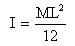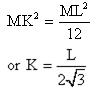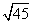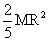Courses

# Test: Moment of Inertia

## 10 Questions MCQ Test Physics Class 11 | Test: Moment of Inertia

Description
This mock test of Test: Moment of Inertia for JEE helps you for every JEE entrance exam. This contains 10 Multiple Choice Questions for JEE Test: Moment of Inertia (mcq) to study with solutions a complete question bank. The solved questions answers in this Test: Moment of Inertia quiz give you a good mix of easy questions and tough questions. JEE students definitely take this Test: Moment of Inertia exercise for a better result in the exam. You can find other Test: Moment of Inertia extra questions, long questions & short questions for JEE on EduRev as well by searching above.
QUESTION: 1

### The radius of gyration of a ring of radius R about an axis through its centre and perpendicular to its plane is

Solution:

For a ring moment of inertia ,I=M*R*R   M=Mass and R=Radius

In terms of radius of gyration

I=M*K*K

M*R*R=M*K*K

Therefore ,K=R

QUESTION: 2

### Two rings have their moment of inertia in the ratio 2:1 and their diameters are in the ratio 2:1. The ratio of their masses will be:

Solution:

We know that MI of a ring is mr2
Where m is mass of the ring and r is its radius
When we have ratio of I = 2:1
And ratio of r = 2:1
We get ratio of r2 = 4:1
Thus to make this ratio 2:1 , that ratio of masses must be 1:2

QUESTION: 3

### The radius of gyration of uniform rod of length L and mass M about an axis passing through its centre and perpendicular to its length is

Solution:

If K is radius of gyration, then moment of inertia for the rod is given as -

I = MK2                        (1)

But moment of inertia of uniform rod of length L and Mass M about an axis passing through its center the perpendicular to its length  is given as -comparing equation (1) and (2), we getQUESTION: 4

Moment of inertia of two spheres of equal radii are equal. One of the spheres is solid and has the mass 5 kg and the other is a hollow sphere. What is the mass of hollow sphere?

Solution:

We know that for a solid sphere of mass S and radius r, moment of inertia I = ⅖ Sr2
Similarly for a hollow sphere of mass H and radius r, moment of inertia I = ⅔ Hr
Thus if there radii and I are equal the ratio of there mass can be find by equating both as
I =  ⅖ Sr2  = ⅔ Hr2
Hence we het H/S = ⅗
So if S is 5kg we get H = 3kg

QUESTION: 5

A solid cylinder of 200g and radius 10 cm has a moment of inertia (about its natural axis)

Solution:

Moment of inertia of solid cylinder
MR2/2=0.2*10*10-2*10*10-2  Kg m2
=2*10-3/2
=10-3 Kg m2

QUESTION: 6

There are two circular iron discs A and B having masses in the ratio 1:2 and diameter in the ratio 2:1. The ratio of their moment of inertia is

Solution:

Given,
Mass of A=1,
Mass of B=2.
diameter if A=2,
diameter if B=1.
radius (r) of A=d/2=2/2=1.
radius (r) of B=d/2=1/2.
we know ,
moment of inertia of disc=MR2/2.
moment of inertia (I)of A/moment of inertia (I)of B=MR2/2/MR2/2.
(I) of A/(I) of B=1×12/2/2×(1/2)2/2.
=1×1/2/2×(1/4)/2.
=1/2/(1/2)/2.
=1/2/1/4.
=4/2.
=2/1.

QUESTION: 7

The moment of inertia of two spheres of equal masses is equal. If one of the spheres is solid of radiusm and the other is a hollow sphere. What is the radius of the hollow sphere?

Solution:

Moment of inertia of solid sphere Is= 2/5MR2
moment of inertia of hollow sphere Ih =2/3MR2
given mass of solid sphere =√45 kg.
Is=Ih
2MR2/5=2MR2/3
given their masses are equal 2 (√45)2/5= 2 R2/3
45/5=R2/3
9=R2/3
9×3=R2
27=R2
√27=R
√3×9=R
3√3 m=R.

QUESTION: 8

The moment of inertia of a body is independent of

Solution:

The moment of inertia of a body is independent of. The moment of inertia of a body is independent of its angular velocity.It depends upon the position and orientation of the axis of rotation,shape and size of the body and distribution of mass of the body about the axis of rotation.

QUESTION: 9

The moment of inertia of a solid sphere about its diameter is.Find moment of inertia about its tangent.

Solution:

The moment of inertia (M.I.) of a sphere about its diameter=2MR2/5
According to the theorem of parallel axes, the moment of inertia of a body about any axis is equal to the sum of the moment of inertia of the body about a parallel axis passing through its centre of mass and the product of its mass and the square of the distance between the two parallel axes.
The M.I. about a tangent of the sphere =2MR2/5+MR2=7MR2/5

QUESTION: 10

A ring has greater moment of inertia than a circular disc of same mass and radius, about an axis passing through its centre of mass perpendicular to its plane, because

Solution:

A ring has a larger moment of inertia because its entire mass is concentrated at the rim at maximum distance from the axis.